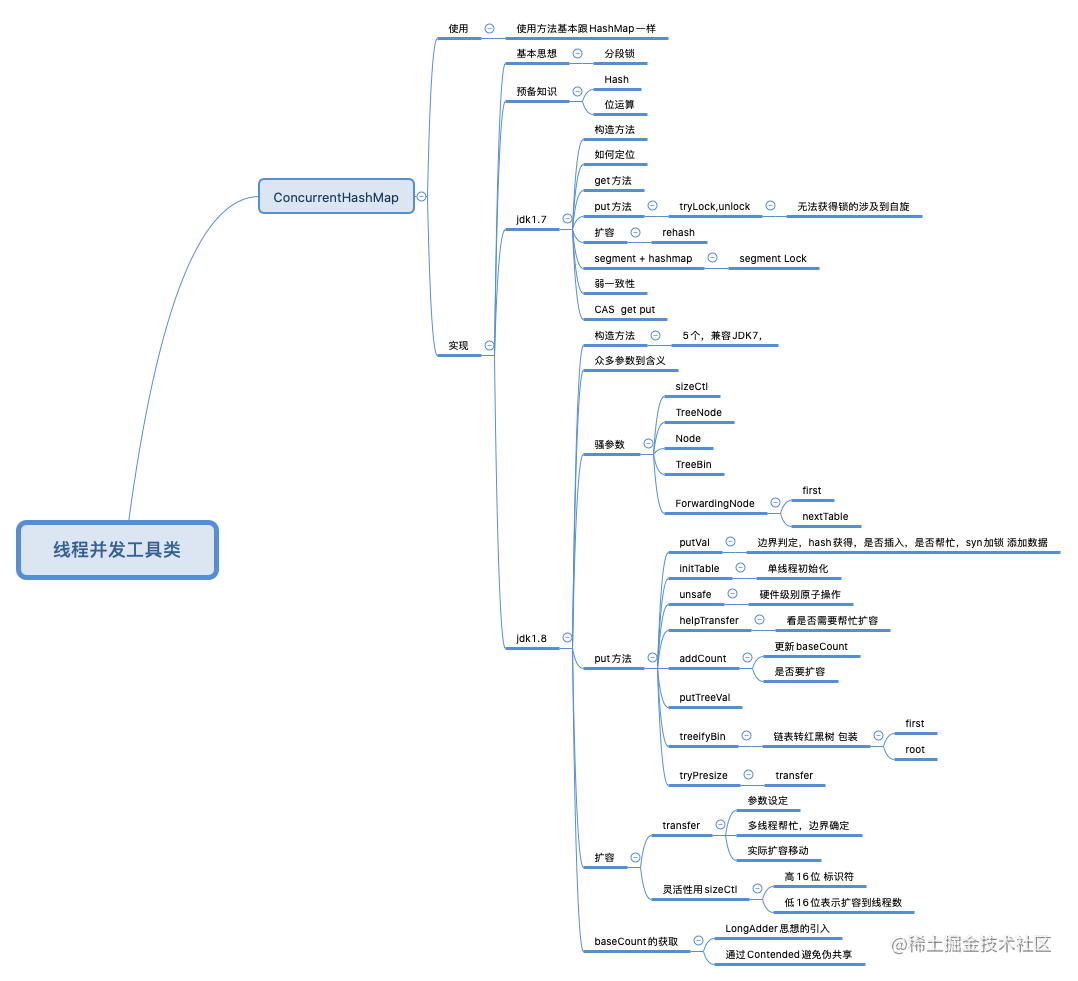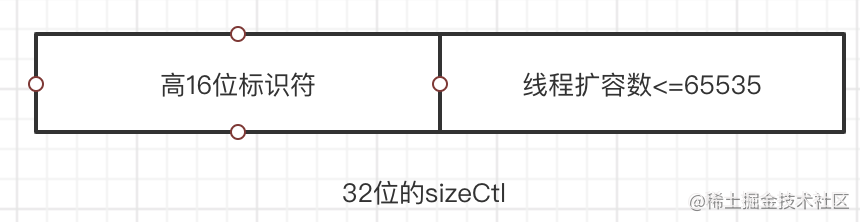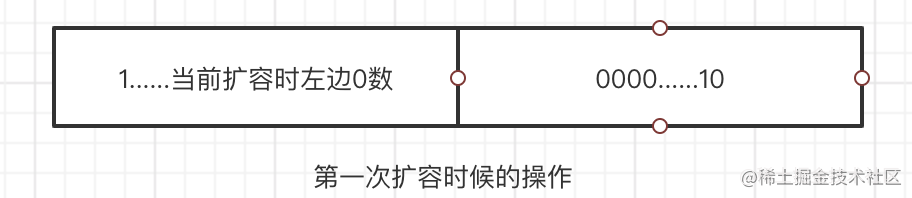# 让ConcurrentHashMap成为你的面试加分点因为上篇文章HashMap已经讲解的很详细了，因此此篇文章会简单介绍思路，再学习并发HashMap就简单很多了，上一篇文章中我们最终知道`HashMap`是线程不安全的，因此在老版本JDK中提供了`HashTable`来实现多线程级别的，改变之处重要有以下几点。

1. `HashTable``put`, `get`,`remove`等方法是通过`synchronized`来修饰保证其线程安全性的。
2. `HashTable`是 不允许key跟value为null的。
3. 问题是`synchronized`是个关键字级别的==重量锁==，在get数据的时候任何写入操作都不允许。相对来说性能不好。因此目前主要用的`ConcurrentHashMap`来保证线程安全性。

`ConcurrentHashMap`主要分为JDK<=7跟JDK>=8的两个版本，`ConcurrentHashMap`的空间利用率更低一般只有10%～20%，接下来分别介绍。

# JDK7

• Segment 是一种可重入锁`ReentrantLock`的子类 ，在 `ConcurrentHashMap` 里扮演锁的角色，`HashEntry`则用于存储键值对数据。
• `ConcurrentHashMap` 里包含一个 `Segment` 数组来实现锁分离，`Segment`的结构和 `HashMap` 类似，一个 `Segment`里包含一个 `HashEntry` 数组，每个 `HashEntry` 是一个链表结构的元素， 每个 `Segment`守护者一个 `HashEntry` 数组里的元素，当对 `HashEntry`数组的数据进行修改时，必须首先获得它对应的 `Segment` 锁。
1. 我们先看下segment类：
``````static final class Segment<K,V> extends ReentrantLock implements Serializable {
transient volatile HashEntry<K,V>[] table; //包含一个HashMap 可以理解为
}

1. 我们看下`currentHashMap`的构造函数，先总结几点。

1. segment的数组大小最终一定是2的次幂
2. 每一个segment里面包含的table(HashEntry数组)初始化大小也一定是2的次幂
3. 这里设置了若干个用于位计算的参数。
4. initialCapacity：初始容量大小 ，默认16。
6. concurrencyLevel:并发度，默认16。并发度可以理解为程序运行时能够同时更新ConccurentHashMap且不产生锁竞争的最大线程数，实际上就是ConcurrentHashMap中的分段锁个数，即Segment[]的数组长度。如果并发度设置的过小，会带来严重的锁竞争问题；如果并发度设置的过大，原本位于同一个Segment内的访问会扩散到不同的Segment中，CPU cache命中率会下降，从而引起程序性能下降。

``````   //initialCapacity 是我们保存所以KV数据的初始值
// 我们segment数组的初始化大小
@SuppressWarnings("unchecked")
public ConcurrentHashMap(int initialCapacity,
if (!(loadFactor > 0) || initialCapacity < 0 || concurrencyLevel <= 0)
throw new IllegalArgumentException();
if (concurrencyLevel > MAX_SEGMENTS) // 最大允许segment的个数，不能超过 1< 24
concurrencyLevel = MAX_SEGMENTS;
int sshift = 0; // 类似扰动函数
int ssize = 1;
while (ssize < concurrencyLevel) {
++sshift;
ssize <<= 1; // 确保segment一定是2次幂
}
this.segmentShift = 32 - sshift;
//有点类似与扰动函数，跟下面的参数配合使用实现 当前元素落到那个segment上面。
this.segmentMask = ssize - 1; // 为了 取模 专用
if (initialCapacity > MAXIMUM_CAPACITY) //不能大于 1< 30
initialCapacity = MAXIMUM_CAPACITY;

int c = initialCapacity / ssize; //总的数组大小 被 segment 分散后 需要多少个table
if (c * ssize < initialCapacity)
++c; //确保向上取值
int cap = MIN_SEGMENT_TABLE_CAPACITY;
// 每个table初始化大小为2
while (cap < c) // 单独的一个segment[i] 对应的table 容量大小。
cap <<= 1;
// 将table的容量初始化为2的次幂
Segment<K,V> s0 =
// 负载因子，阈值，每个segment的初始化大小。跟hashmap 初始值类似。
// 并且segment的初始化是懒加载模式，刚开始只有一个s0，其余的在需要的时候才会增加。
Segment<K,V>[] ss = (Segment<K,V>[])new Segment[ssize];
UNSAFE.putOrderedObject(ss, SBASE, s0); // ordered write of segments
this.segments = ss;
}

1. hash 不管是我们的get操作还是put操作要需要通过hash来对数据进行定位。
``````   //  整体思想就是通过多次不同方式的位运算来努力将数据均匀的分不到目标table中，都是些扰动函数
private int hash(Object k) {
int h = hashSeed;
if ((0 != h) && (k instanceof String)) {
return sun.misc.Hashing.stringHash32((String) k);
}
h ^= k.hashCode();
// single-word Wang/Jenkins hash.
h += (h <<  15) ^ 0xffffcd7d;
h ^= (h >>> 10);
h += (h <<   3);
h ^= (h >>>  6);
h += (h <<   2) + (h << 14);
return h ^ (h >>> 16);
}

1. get 相对来说比较简单，无非就是通过`hash`找到对应的`segment`，继续通过`hash`找到对应的`table`,然后就是遍历这个链表看是否可以找到，并且要注意 `get`的时候是==没有加锁==的。
``````   public V get(Object key) {
Segment<K,V> s;
HashEntry<K,V>[] tab;
int h = hash(key); // JDK7中标准的hash值获取算法
long u = (((h >>> segmentShift) & segmentMask) << SSHIFT) + SBASE; // hash值如何映射到对应的segment上
if ((s = (Segment<K,V>)UNSAFE.getObjectVolatile(segments, u)) != null && (tab = s.table) != null) {
//  无非就是获得hash值对应的segment 是否存在，
for (HashEntry<K,V> e = (HashEntry<K,V>) UNSAFE.getObjectVolatile
(tab, ((long)(((tab.length - 1) & h)) << TSHIFT) + TBASE);
e != null; e = e.next) {
// 看下这个hash值对应的是segment(HashEntry)中的具体位置。然后遍历查询该链表
K k;
if ((k = e.key) == key || (e.hash == h && key.equals(k)))
return e.value;
}
}
return null;
}

1. put 相同的思路，先找到`hash`值对应的`segment`位置，然后看该`segment`位置是否初始化了(因为segment是==懒加载==模式)。选择性初始化，最终执行put操作。
``````   @SuppressWarnings("unchecked")
public V put(K key, V value) {
Segment<K,V> s;
if (value == null)
throw new NullPointerException();
int hash = hash(key);// 还是获得最终hash值
int j = (hash >>> segmentShift) & segmentMask; // hash值位操作对应的segment数组位置
if ((s = (Segment<K,V>)UNSAFE.getObject
(segments, (j << SSHIFT) + SBASE)) == null)
s = ensureSegment(j);
// 初始化时候因为只有第一个segment，如果落在了其余的segment中 则需要现初始化。
return s.put(key, hash, value, false);
// 直接在数据中执行put操作。
}

``````final V put(K key, int hash, V value, boolean onlyIfAbsent) {
// 在往该 segment 写入前，需要先获取该 segment 的独占锁，获取失败尝试获取自旋锁
HashEntry<K,V> node = tryLock() ? null :
scanAndLockForPut(key, hash, value);
V oldValue;
try {
// segment 内部的数组
HashEntry<K,V>[] tab = table;
// 利用 hash 值，求应该放置的数组下标
int index = (tab.length - 1) & hash;
// first 是数组该位置处的链表的表头
HashEntry<K,V> first = entryAt(tab, index);

for (HashEntry<K,V> e = first;;) {
if (e != null) {
K k;
if ((k = e.key) == key ||
(e.hash == hash && key.equals(k))) {
oldValue = e.value;
if (!onlyIfAbsent) {
// 覆盖旧值
e.value = value;
++modCount;
}
break;
}
// 继续顺着链表走
e = e.next;
}
else {
// node 是不是 null，这个要看获取锁的过程。没获得锁的线程帮我们创建好了节点，直接头插法
// 如果不为 null，那就直接将它设置为链表表头；如果是 null，初始化并设置为链表表头。
if (node != null)
node.setNext(first);
else
node = new HashEntry<K,V>(hash, key, value, first);

int c = count + 1;
// 如果超过了该 segment 的阈值，这个 segment 需要扩容
if (c > threshold && tab.length < MAXIMUM_CAPACITY)
rehash(node); // 扩容
else
// 没有达到阈值，将 node 放到数组 tab 的 index 位置，
// 将新的结点设置成原链表的表头
setEntryAt(tab, index, node);
++modCount;
count = c;
oldValue = null;
break;
}
}
} finally {
// 解锁
unlock();
}
return oldValue;
}

1. 在我获取不到锁的时候我进行tryLock,准备好new的数据，同时还有一定的次数限制，还要考虑别的已经获得线程的节点修改该头节点。
``````private HashEntry<K,V> scanAndLockForPut(K key, int hash, V value) {
HashEntry<K,V> first = entryForHash(this, hash);
HashEntry<K,V> e = first;
HashEntry<K,V> node = null;
int retries = -1; // negative while locating node

// 循环获取锁
while (!tryLock()) {
HashEntry<K,V> f; // to recheck first below
if (retries < 0) {
if (e == null) {
if (node == null) // speculatively create node
// 进到这里说明数组该位置的链表是空的，没有任何元素
// 当然，进到这里的另一个原因是 tryLock() 失败，所以该槽存在并发，不一定是该位置
node = new HashEntry<K,V>(hash, key, value, null);
retries = 0;
}
else if (key.equals(e.key))
retries = 0;
else
// 顺着链表往下走
e = e.next;
}
// 重试次数如果超过 MAX_SCAN_RETRIES（单核 1 次多核 64 次），那么不抢了，进入到阻塞队列等待锁
//    lock() 是阻塞方法，直到获取锁后返回
else if (++retries > MAX_SCAN_RETRIES) {
lock();
break;
}
else if ((retries & 1) == 0 &&
// 进入这里，说明有新的元素进到了链表，并且成为了新的表头
// 这边的策略是，重新执行 scanAndLockForPut 方法
(f = entryForHash(this, hash)) != first) {
e = first = f; // re-traverse if entry changed
retries = -1;
}
}
return node;
}

1. Size

这个size方法比较有趣，他是先无锁的统计下所有的数据量看下前后两次是否数据一样，如果一样则返回数据，如果不一样则要把全部的segment进行加锁，统计，解锁。并且size方法只是返回一个统计性的数字，因此size谨慎使用哦。

``````public int size() {
// Try a few times to get accurate count. On failure due to
// continuous async changes in table, resort to locking.
final Segment<K,V>[] segments = this.segments;
int size;
boolean overflow; // true if size overflows 32 bits
long sum;         // sum of modCounts
long last = 0L;   // previous sum
int retries = -1; // first iteration isn't retry
try {
for (;;) {
if (retries++ == RETRIES_BEFORE_LOCK) {  //  超过2次则全部加锁
for (int j = 0; j < segments.length; ++j)
ensureSegment(j).lock(); // 直接对全部segment加锁消耗性太大
}
sum = 0L;
size = 0;
overflow = false;
for (int j = 0; j < segments.length; ++j) {
Segment<K,V> seg = segmentAt(segments, j);
if (seg != null) {
sum += seg.modCount; // 统计的是modCount,涉及到增删该都会加1
int c = seg.count;
if (c < 0 || (size += c) < 0)
overflow = true;
}
}
if (sum == last) // 每一个前后的修改次数一样 则认为一样，但凡有一个不一样则直接break。
break;
last = sum;
}
} finally {
if (retries > RETRIES_BEFORE_LOCK) {
for (int j = 0; j < segments.length; ++j)
segmentAt(segments, j).unlock();
}
}
return overflow ? Integer.MAX_VALUE : size;
}

1. rehash `segment` 数组初始化后就不可变了，也就是说并发性不可变，不过`segment`里的`table`可以扩容为2倍，该方法没有考虑并发，因为执行该方法之前已经获取了锁。其中JDK7中的`rehash`思路跟JDK8 中扩容后处理链表的思路一样，个人不过感觉没有8写的精髓好看。
``````// 方法参数上的 node 是这次扩容后，需要添加到新的数组中的数据。
private void rehash(HashEntry<K,V> node) {
HashEntry<K,V>[] oldTable = table;
int oldCapacity = oldTable.length;
// 2 倍
int newCapacity = oldCapacity << 1;
// 创建新数组
HashEntry<K,V>[] newTable =
(HashEntry<K,V>[]) new HashEntry[newCapacity];
// 新的掩码，如从 16 扩容到 32，那么 sizeMask 为 31，对应二进制 ‘000...00011111’
int sizeMask = newCapacity - 1;
// 遍历原数组，将原数组位置 i 处的链表拆分到 新数组位置 i 和 i+oldCap 两个位置
for (int i = 0; i < oldCapacity ; i++) {
// e 是链表的第一个元素
HashEntry<K,V> e = oldTable[i];
if (e != null) {
HashEntry<K,V> next = e.next;
// 计算应该放置在新数组中的位置，
// 假设原数组长度为 16，e 在 oldTable 处，那么 idx 只可能是 3 或者是 3 + 16 = 19
int idx = e.hash & sizeMask; // 新位置
if (next == null)   // 该位置处只有一个元素
newTable[idx] = e;
else { // Reuse consecutive sequence at same slot
// e 是链表表头
HashEntry<K,V> lastRun = e;
// idx 是当前链表的头结点 e 的新位置
int lastIdx = idx;
// for 循环找到一个 lastRun 结点，这个结点之后的所有元素是将要放到一起的
for (HashEntry<K,V> last = next;
last != null;
last = last.next) {
int k = last.hash & sizeMask;
if (k != lastIdx) {
lastIdx = k;
lastRun = last;
}
}
// 将 lastRun 及其之后的所有结点组成的这个链表放到 lastIdx 这个位置
newTable[lastIdx] = lastRun;
// 下面的操作是处理 lastRun 之前的结点，
//这些结点可能分配在另一个链表中，也可能分配到上面的那个链表中
for (HashEntry<K,V> p = e; p != lastRun; p = p.next) {
V v = p.value;
int h = p.hash;
int k = h & sizeMask;
HashEntry<K,V> n = newTable[k];
newTable[k] = new HashEntry<K,V>(h, p.key, v, n);
}
}
}
}
// 将新来的 node 放到新数组中刚刚的 两个链表之一 的 头部
node.setNext(newTable[nodeIndex]);
newTable[nodeIndex] = node;
table = newTable;
}

1. CAS操作 在JDK7里在`ConcurrentHashMap`中通过原子操作`sun.misc.Unsafe`查找元素、替换元素和设置元素。通过这样的硬件级别获得数据可以保证及时是多线程我也每次获得的数据是最新的。这些原子操作起着非常关键的作用，你可以在所有`ConcurrentHashMap`的基本功能中看到，随机距离如下：
``````     final void setNext(HashEntry<K,V> n) {
UNSAFE.putOrderedObject(this, nextOffset, n);
}
static final <K,V> HashEntry<K,V> entryAt(HashEntry<K,V>[] tab, int i) {
return (tab == null) ? null :
(HashEntry<K,V>) UNSAFE.getObjectVolatile
(tab, ((long)i << TSHIFT) + TBASE);
}
static final <K,V> void setEntryAt(HashEntry<K,V>[] tab, int i,
HashEntry<K,V> e) {
UNSAFE.putOrderedObject(tab, ((long)i << TSHIFT) + TBASE, e);
}

### 常见问题

1. ConcurrentHashMap实现原理是怎么样的或者ConcurrentHashMap如何在保证高并发下线程安全的同时实现了性能提升？

`ConcurrentHashMap`允许多个修改操作并发进行，其关键在于使用了==锁分离==技术。它使用了多个锁来控制对hash表的不同部分进行的修改。内部使用段(`Segment`)来表示这些不同的部分，每个段其实就是一个小的`HashTable`，只要多个修改操作发生在不同的段上，它们就可以并发进行。

1. 在高并发下的情况下如何保证取得的元素是最新的？

1. ConcurrentHashMap的弱一致性体现在迭代器,clear和get方法，原因在于没有加锁。

1. 比如迭代器在遍历数据的时候是一个Segment一个Segment去遍历的，如果在遍历完一个Segment时正好有一个线程在刚遍历完的Segment上插入数据，就会体现出不一致性。clear也是一样。
2. get方法和containsKey方法都是遍历对应索引位上所有节点，都是不加锁来判断的，如果是修改性质的因为可见性的存在可以直接获得最新值，不过如果是新添加值则无法保持一致性

# JDK8

JDK8相比与JDK7主要区别如下：

1. 取消了segment数组，直接用table保存数据，锁的粒度更小，减少并发冲突的概率。采用table数组元素作为锁，从而实现了对每一行数据进行加锁，进一步减少并发冲突的概率，并发控制使用Synchronized和CAS来操作。
2. 存储数据时采用了数组+ 链表+红黑树的形式。
1. CurrentHashMap重要参数：

private static final int MAXIMUM_CAPACITY = 1 << 30; // 数组的最大值 private static final int DEFAULT_CAPACITY = 16; // 默认数组长度 static final int TREEIFY_THRESHOLD = 8; // 链表转红黑树的一个条件 static final int UNTREEIFY_THRESHOLD = 6; // 红黑树转链表的一个条件 static final int MIN_TREEIFY_CAPACITY = 64; // 链表转红黑树的另一个条件 static final int MOVED = -1; // 表示正在扩容转移 static final int TREEBIN = -2; // 表示已经转换成树 static final int RESERVED = -3; // hash for transient reservations static final int HASH_BITS = 0x7fffffff; // 获得hash值的辅助参数 transient volatile Node<K,V>[] table;// 默认没初始化的数组，用来保存元素 private transient volatile Node<K,V>[] nextTable; // 转移的时候用的数组 static final int NCPU = Runtime.getRuntime().availableProcessors();// 获取可用的CPU个数 private transient volatile Node<K,V>[] nextTable; // 连接表，用于哈希表扩容，扩容完成后会被重置为 null private transient volatile long baseCount;保存着整个哈希表中存储的所有的结点的个数总和，有点类似于 HashMap 的 size 属性。 private transient volatile int `sizeCtl`; 负数：表示进行初始化或者扩容，-1：表示正在初始化，-N：表示有 N-1 个线程正在进行扩容 正数：0 表示还没有被初始化，> 0的数：初始化或者是下一次进行扩容的阈值，有点类似HashMap中的`threshold`，不过功能更强大

1. 若干重要类
• 构成每个元素的基本类 `Node`
``````      static class Node<K,V> implements Map.Entry<K,V> {
final int hash;    // key的hash值
final K key;       // key
volatile V val;    // value
volatile Node<K,V> next;
//表示链表中的下一个节点
}

• TreeNode继承于Node，用来存储红黑树节点
``````      static final class TreeNode<K,V> extends Node<K,V> {
TreeNode<K,V> parent;
// 红黑树的父亲节点
TreeNode<K,V> left;
// 左节点
TreeNode<K,V> right;
// 右节点
TreeNode<K,V> prev;
// 前节点
boolean red;
// 是否为红点
}

• ForwardingNode 在 Node 的子类 `ForwardingNode` 的构造方法中，可以看到此变量的hash = -1 ，类中还存储`nextTable`的引用。该初始化方法只在 `transfer`方法被调用，如果一个类被设置成此种情况并且hash = -1 则说明该节点不需要resize了。
``````static final class ForwardingNode<K,V> extends Node<K,V> {
final Node<K,V>[] nextTable;
ForwardingNode(Node<K,V>[] tab) {
//注意这里
super(MOVED, null, null, null);
this.nextTable = tab;
}
//.....
}

• TreeBin TreeBin从字面含义中可以理解为存储树形结构的容器，而树形结构就是指TreeNode，所以TreeBin就是封装TreeNode的容器，它提供转换黑红树的一些条件和锁的控制.
``````static final class TreeBin<K,V> extends Node<K,V> {
TreeNode<K,V> root;
volatile TreeNode<K,V> first;
volatile int lockState;
// values for lockState
static final int WRITER = 1; // set while holding write lock
static final int WAITER = 2; // set when waiting for write lock
static final int READER = 4; // increment value for setting read lock
}

### 构造函数

1. ConcurrentHashMap()： 创建一个带有默认初始容量 (16)、加载因子 (0.75) 和 concurrencyLevel (16) 的新的空映射
2. ConcurrentHashMap(int)：创建一个带有指定初始容量`tableSizeFor`、默认加载因子 (0.75) 和 concurrencyLevel (16) 的新的空映射
3. ConcurrentHashMap(Map<? extends K, ? extends V> m)：构造一个与给定映射具有相同映射关系的新映射
4. ConcurrentHashMap(int initialCapacity, float loadFactor)：创建一个带有指定初始容量、加载因子和默认 concurrencyLevel (1) 的新的空映射
5. ConcurrentHashMap(int, float, int)：创建一个带有指定初始容量、加载因子和并发级别的新的空映射。

### put

1. 做一些边界处理，然后获得hash值。
2. 没初始化就初始化，初始化后看下对应的桶是否为空，为空就原子性的尝试插入。
3. 如果当前节点正在扩容还要去帮忙扩容，骚操作。
4. `syn`来加锁当前节点，然后操作几乎跟就跟hashmap一样了。
``````
// Node 节点的 hash值在HashMap中存储的就是hash值，在currenthashmap中可能有多种情况哦！
final V putVal(K key, V value, boolean onlyIfAbsent) {
if (key == null || value == null) throw new NullPointerException(); //边界处理
int binCount = 0;
for (Node<K,V>[] tab = table;;) { //循环表
Node<K,V> f; int n, i, fh;
if (tab == null || (n = tab.length) == 0)
tab = initTable(); // 初始化表 如果为空,懒汉式
else if ((f = tabAt(tab, i = (n - 1) & hash)) == null) {
// 如果对应桶位置为空
if (casTabAt(tab, i, null, new Node<K,V>(hash, key, value, null)))
// CAS 原子性的尝试插入
break;
}
else if ((fh = f.hash) == MOVED)
// 如果当前节点正在扩容。还要帮着去扩容。
tab = helpTransfer(tab, f);
else {
V oldVal = null;
synchronized (f) { //  桶存在数据 加锁操作进行处理
if (tabAt(tab, i) == f) {
if (fh >= 0) { // 如果存储的是链表 存储的是节点的hash值
binCount = 1;
for (Node<K,V> e = f;; ++binCount) {
K ek;
// 遍历链表去查找，如果找到key一样则选择性
if (e.hash == hash &&
((ek = e.key) == key ||
(ek != null && key.equals(ek)))) {
oldVal = e.val;
if (!onlyIfAbsent)
e.val = value;
break;
}
Node<K,V> pred = e;
if ((e = e.next) == null) {// 找到尾部插入
pred.next = new Node<K,V>(hash, key,
value, null);
break;
}
}
}
else if (f instanceof TreeBin) {// 如果桶节点类型为TreeBin
Node<K,V> p;
binCount = 2;
if ((p = ((TreeBin<K,V>)f).putTreeVal(hash, key,
value)) != null) {
// 尝试红黑树插入，同时也要防止节点本来就有，选择性覆盖
oldVal = p.val;
if (!onlyIfAbsent)
p.val = value;
}
}
}
}
if (binCount != 0) { // 如果链表数量
if (binCount >= TREEIFY_THRESHOLD)
treeifyBin(tab, i); //  链表转红黑树哦！
if (oldVal != null)
return oldVal;
break;
}
}
}
return null;
}

### initTable

``````// 容器初始化 操作
private final Node<K,V>[] initTable() {
Node<K,V>[] tab; int sc;
while ((tab = table) == null || tab.length == 0) {
if ((sc = sizeCtl) < 0) // 如果正在初始化-1，-N 正在扩容。
// 让掉当前线程 CPU 的时间片，使正在运行中的线程重新变成就绪状态，并重新竞争 CPU 的调度权。
// 它可能会获取到，也有可能被其他线程获取到。
else if (U.compareAndSwapInt(this, SIZECTL, sc, -1)) {
//  比较sizeCtl的值与sc是否相等，相等则用 -1 替换,这表明我这个线程在进行初始化了！
try {
if ((tab = table) == null || tab.length == 0) {
int n = (sc > 0) ? sc : DEFAULT_CAPACITY; // 默认为16
@SuppressWarnings("unchecked")
Node<K,V>[] nt = (Node<K,V>[])new Node<?,?>[n];
table = tab = nt;
sc = n - (n >>> 2); // sc = 0.75n
}
} finally {
sizeCtl = sc; //设置sizeCtl 类似threshold
}
break;
}
}
return tab;
}

### unsafe

`ConcurrentHashMap`中使用了`unSafe`方法，通过直接操作内存的方式来保证并发处理的安全性，使用的是==硬件==的安全机制。

`````` // 用来返回节点数组的指定位置的节点的原子操作
@SuppressWarnings("unchecked")
static final <K,V> Node<K,V> tabAt(Node<K,V>[] tab, int i) {
return (Node<K,V>)U.getObjectVolatile(tab, ((long)i << ASHIFT) + ABASE);
}

// cas原子操作，在指定位置设定值
static final <K,V> boolean casTabAt(Node<K,V>[] tab, int i,
Node<K,V> c, Node<K,V> v) {
return U.compareAndSwapObject(tab, ((long)i << ASHIFT) + ABASE, c, v);
}

// 原子操作，在指定位置设定值
static final <K,V> void setTabAt(Node<K,V>[] tab, int i, Node<K,V> v) {
U.putObjectVolatile(tab, ((long)i << ASHIFT) + ABASE, v);
}

// 比较table数组下标为i的结点是否为c，若为c，则用v交换操作。否则，不进行交换操作。
static final <K,V> boolean casTabAt(Node<K,V>[] tab, int i,
Node<K,V> c, Node<K,V> v) {
return U.compareAndSwapObject(tab, ((long)i << ASHIFT) + ABASE, c, v);
}

### helpTransfer

``````// 可能有多个线程在同时帮忙运行helpTransfer
final Node<K,V>[] helpTransfer(Node<K,V>[] tab, Node<K,V> f) {
Node<K,V>[] nextTab; int sc;
if (tab != null && (f instanceof ForwardingNode) && (nextTab = ((ForwardingNode<K,V>)f).nextTable) != null) {
// table不是空  且 node节点是转移类型，并且转移类型的nextTable 不是空 说明还在扩容ing
int rs = resizeStamp(tab.length);
// 根据 length 得到一个前16位的标识符，数组容量大小。
// 确定新table指向没有变，老table数据也没变，并且此时 sizeCtl小于0 还在扩容ing
while (nextTab == nextTable && table == tab && (sc = sizeCtl) < 0) {
if ((sc >>> RESIZE_STAMP_SHIFT) != rs || sc == rs + 1 || sc == rs + MAX_RESIZERS || transferIndex <= 0)
// 1. sizeCtl 无符号右移16位获得高16位如果不等 rs 标识符变了
// 2. 如果扩容结束了 这里可以看 trePresize 函数第一次扩容操作：
// 默认第一个线程设置 sc = rs 左移 16 位 + 2，当第一个线程结束扩容了，
// 就会将 sc 减一。这个时候，sc 就等于 rs + 1。
// 3. 如果达到了最大帮助线程个数 65535个
// 4. 如果转移下标调整ing 扩容已经结束了
break;
if (U.compareAndSwapInt(this, SIZECTL, sc, sc + 1)) {
// 如果以上都不是, 将 sizeCtl + 1,增加一个线程来扩容
transfer(tab, nextTab); // 进行转移
break;// 结束循环
}
}
return nextTab;
}
return table;
}

• resizeStamp 主要用来获得标识符，可以简单理解是对当前系统容量大小的一种监控。
``````static final int resizeStamp(int n) {
return Integer.numberOfLeadingZeros(n) | (1 << (RESIZE_STAMP_BITS - 1));
//RESIZE_STAMP_BITS = 16
}

``````private final void addCount(long x, int check) {
CounterCell[] as; long b, s;
// 首先如果没有并发 此时countCells is null, 此时尝试CAS设置数据值。
if ((as = counterCells) != null || !U.compareAndSwapLong(this, BASECOUNT, b = baseCount, s = b + x)) {
// 如果 counterCells不为空以为此时有并发的设置 或者 CAS设置 baseCount 失败了
CounterCell a; long v; int m;
boolean uncontended = true;
if (as == null || (m = as.length - 1) < 0 || (a = as[ThreadLocalRandom.getProbe() & m]) == null ||
!(uncontended = U.compareAndSwapLong(a, CELLVALUE, v = a.value, v + x))) {
// 1. 如果没出现并发 此时计数盒子为 null
// 2. 随机取出一个数组位置发现为空
// 3. 出现并发后修改这个cellvalue 失败了
return;
}
if (check <= 1)
return;
s = sumCount(); // 吧counterCells数组中的每一个数据进行累加给baseCount。
}
// 如果需要扩容
if (check >= 0) {
Node<K,V>[] tab, nt; int n, sc;
while (s >= (long)(sc = sizeCtl) && (tab = table) != null && (n = tab.length) < MAXIMUM_CAPACITY) {
int rs = resizeStamp(n);// 获得高位标识符
if (sc < 0) { // 是否需要帮忙去扩容
if ((sc >>> RESIZE_STAMP_SHIFT) != rs || sc == rs + 1 ||
sc == rs + MAX_RESIZERS || (nt = nextTable) == null || transferIndex <= 0)
break;
if (U.compareAndSwapInt(this, SIZECTL, sc, sc + 1))
transfer(tab, nt);
} // 第一次扩容
else if (U.compareAndSwapInt(this, SIZECTL, sc, (rs << RESIZE_STAMP_SHIFT) + 2))
transfer(tab, null);
s = sumCount();
}
}
}

1. baseCount添加 `ConcurrentHashMap`提供了`baseCount``counterCells` 两个辅助变量和一个 `CounterCell`辅助内部类。sumCount() 就是迭代 `counterCells`来统计 sum 的过程。 put 操作时，肯定会影响 `size()`，在 `put()` 方法最后会调用 `addCount()`方法。整体的思维方法跟LongAdder类似，用的思维就是借鉴的`ConcurrentHashMap`。每一个`Cell`都用Contended修饰来避免伪共享。
1. JDK1.7 和 JDK1.8 对 size 的计算是不一样的。 1.7 中是先不加锁计算三次，如果三次结果不一样在加锁。
2. JDK1.8 size 是通过对 baseCount 和 counterCell 进行 CAS 计算，最终通过 baseCount 和 遍历 CounterCell 数组得出 size。
3. JDK 8 推荐使用mappingCount 方法，因为这个方法的返回值是 long 类型，不会因为 size 方法是 int 类型限制最大值。
1. 关于扩容`addCount`第一次扩容时候会有骚操作`sc=rs << RESIZE_STAMP_SHIFT) + 2)`其中`rs = resizeStamp(n)`。这里需要核心说一点，如果不是第一次扩容则直接将低16位的数字 +1 即可。

### treeifyBin

``````private final void treeifyBin(Node<K,V>[] tab, int index) {
Node<K,V> b; int n, sc;
if (tab != null) {
//如果整个table的数量小于64，就扩容至原来的一倍，不转红黑树了
//因为这个阈值扩容可以减少hash冲突，不必要去转红黑树
if ((n = tab.length) < MIN_TREEIFY_CAPACITY)
tryPresize(n << 1);
else if ((b = tabAt(tab, index)) != null && b.hash >= 0) {
synchronized (b) { //锁定当前桶
if (tabAt(tab, index) == b) {
TreeNode<K,V> hd = null, tl = null;
for (Node<K,V> e = b; e != null; e = e.next) {
//遍历这个链表然后将每个节点封装成TreeNode，最终单链表串联起来，
// 最终 调用setTabAt 放置红黑树
TreeNode<K,V> p =
new TreeNode<K,V>(e.hash, e.key, e.val,
null, null);
if ((p.prev = tl) == null)
hd = p;
else
tl.next = p;
tl = p;
}
//通过TreeBin对象对TreeNode转换成红黑树
setTabAt(tab, index, new TreeBin<K,V>(hd));
}
}
}
}
}

### TreeBin

1. TreeBin.first = 链表中第一个节点。
2. TreeBin.root = 红黑树中的root节点。
``````TreeBin(TreeNode<K,V> b) {
super(TREEBIN, null, null, null);
//创建空节点 hash = -2
this.first = b;
TreeNode<K,V> r = null; // root 节点
for (TreeNode<K,V> x = b, next; x != null; x = next) {
next = (TreeNode<K,V>)x.next;
x.left = x.right = null;
if (r == null) {
x.parent = null;
x.red = false;
r = x; // root 节点设置为x
}
else {
K k = x.key;
int h = x.hash;
Class<?> kc = null;
for (TreeNode<K,V> p = r;;) {
// x代表的是转换为树之前的顺序遍历到链表的位置的节点，r代表的是根节点
int dir, ph;
K pk = p.key;
if ((ph = p.hash) > h)
dir = -1;
else if (ph < h)
dir = 1;
else if ((kc == null &&
(kc = comparableClassFor(k)) == null) ||
(dir = compareComparables(kc, k, pk)) == 0)
dir = tieBreakOrder(k, pk);
// 当key不可以比较，或者相等的时候采取的一种排序措施
TreeNode<K,V> xp = p;
// 放一定是放在叶子节点上，如果还没找到叶子节点则进行循环往下找。
// 找到了目前叶子节点才会进入 再放置数据
if ((p = (dir <= 0) ? p.left : p.right) == null) {
x.parent = xp;
if (dir <= 0)
xp.left = x;
else
xp.right = x;
r = balanceInsertion(r, x);
// 每次插入一个元素的时候都调用 balanceInsertion 来保持红黑树的平衡
break;
}
}
}
}
this.root = r;
assert checkInvariants(root);
}

### tryPresize

PS： 默认第一个线程设置 sc = rs 左移 16 位 + 2，当第一个线程结束扩容了，就会将 sc 减一。这个时候，sc 就等于 rs + 1，这个时候说明扩容完毕了。

``````     /**
* 扩容表为指可以容纳指定个数的大小（总是2的N次方）
* 假设原来的数组长度为16，则在调用tryPresize的时候，size参数的值为16<<1(32)，此时sizeCtl的值为12
* 计算出来c的值为64, 则要扩容到 sizeCtl ≥ c
*  第一次扩容之后 数组长：32 sizeCtl：24
*  第三次扩容之后 数组长：128  sizeCtl：96 退出
*/
private final void tryPresize(int size) {
int c = (size >= (MAXIMUM_CAPACITY >>> 1)) ? MAXIMUM_CAPACITY :
tableSizeFor(size + (size >>> 1) + 1); // 合理范围
int sc;
while ((sc = sizeCtl) >= 0) {
Node<K,V>[] tab = table; int n;
if (tab == null || (n = tab.length) == 0) {
// 初始化传入map，今天putAll会直接调用这个。
n = (sc > c) ? sc : c;
if (U.compareAndSwapInt(this, SIZECTL, sc, -1)) {
//初始化tab的时候，把 sizeCtl 设为 -1
try {
if (table == tab) {
@SuppressWarnings("unchecked")
Node<K,V>[] nt = (Node<K,V>[])new Node<?,?>[n];
table = nt;
sc = n - (n >>> 2); // sc=sizeCtl = 0.75n
}
} finally {
sizeCtl = sc;
}
}
}
// 初始化时候如果  数组容量<=sizeCtl 或 容量已经最大化了则退出
else if (c <= sc || n >= MAXIMUM_CAPACITY) {
break;//退出扩张
}
else if (tab == table) {
int rs = resizeStamp(n);

if (sc < 0) { // sc = siztCtl 如果正在扩容Table的话，则帮助扩容
Node<K,V>[] nt;
if ((sc >>> RESIZE_STAMP_SHIFT) != rs || sc == rs + 1 ||
sc == rs + MAX_RESIZERS || (nt = nextTable) == null ||
transferIndex <= 0)
break; // 各种条件判断是否需要加入扩容工作。
// 帮助转移数据的线程数 + 1
if (U.compareAndSwapInt(this, SIZECTL, sc, sc + 1))
transfer(tab, nt);
}
// 没有在初始化或扩容，则开始扩容
// 此处切记第一次扩容 直接 +2
else if (U.compareAndSwapInt(this, SIZECTL, sc,
(rs << RESIZE_STAMP_SHIFT) + 2)) {
transfer(tab, null);
}
}
}
}

### transfer

1. 主要是 单个线程能处理的最少桶结点个数的计算和一些属性的初始化操作。
2. 每个线程进来会先领取自己的任务区间`[bound,i]`，然后开始 --i 来遍历自己的任务区间，对每个桶进行处理。如果遇到桶的头结点是空的，那么使用 `ForwardingNode`标识旧table中该桶已经被处理完成了。如果遇到已经处理完成的桶，直接跳过进行下一个桶的处理。如果是正常的桶，对桶首节点加锁，正常的迁移即可(跟HashMap第三部分一样思路)，迁移结束后依然会将原表的该位置标识位已经处理。

``````f ((sc - 2) != resizeStamp(n) << RESIZE_STAMP_SHIFT)
return;

1. 几乎跟`HashMap`大致思路类似的遍历链表/红黑树然后扩容操作。
``````private final void transfer(Node<K,V>[] tab, Node<K,V>[] nextTab) {
int n = tab.length, stride;
if ((stride = (NCPU > 1) ? (n >>> 3) / NCPU : n) < MIN_TRANSFER_STRIDE)    //MIN_TRANSFER_STRIDE 用来控制不要占用太多CPU
stride = MIN_TRANSFER_STRIDE; // subdivide range    //MIN_TRANSFER_STRIDE=16 每个CPU处理最小长度个数

if (nextTab == null) { // 新表格为空则直接新建二倍，别的辅助线程来帮忙扩容则不会进入此if条件
try {
@SuppressWarnings("unchecked")
Node<K,V>[] nt = (Node<K,V>[])new Node<?,?>[n << 1];
nextTab = nt;
} catch (Throwable ex) {      // try to cope with OOME
sizeCtl = Integer.MAX_VALUE;
return;
}
nextTable = nextTab;
transferIndex = n; // transferIndex 指向最后一个桶，方便从后向前遍历
}
int nextn = nextTab.length; // 新表长度
ForwardingNode<K,V> fwd = new ForwardingNode<K,V>(nextTab); // 创建一个fwd节点，这个是用来控制并发的，当一个节点为空或已经被转移之后，就设置为fwd节点
boolean finishing = false; // to ensure sweep(清扫) before committing nextTab,在完成之前重新在扫描一遍数组，看看有没完成的没
// 第一部分
// i 指向当前桶， bound 指向当前线程需要处理的桶结点的区间下限【bound，i】 这样来跟线程划分任务。
for (int i = 0, bound = 0;;) {
Node<K,V> f; int fh;
// 这个 while 循环的目的就是通过 --i 遍历当前线程所分配到的桶结点
// 一个桶一个桶的处理
int nextIndex, nextBound;
if (--i >= bound || finishing) { //通过此处进行任务区间的遍历
}
else if ((nextIndex = transferIndex) <= 0) {
i = -1;// 任务分配完了
}
// 更新 transferIndex
// 为当前线程分配任务，处理的桶结点区间为（nextBound,nextIndex）
else if (U.compareAndSwapInt(this, TRANSFERINDEX, nextIndex,nextBound = (nextIndex > stride ? nextIndex - stride : 0))) {
// nextIndex本来等于末尾数字，
bound = nextBound;
i = nextIndex - 1;
}
}
// 当前线程所有任务完成
if (i < 0 || i >= n || i + n >= nextn) {
int sc;
if (finishing) {  // 已经完成转移 则直接赋值操作
nextTable = null;
table = nextTab;
sizeCtl = (n << 1) - (n >>> 1);    //设置sizeCtl为扩容后的0.75
return;
}
if (U.compareAndSwapInt(this, SIZECTL, sc = sizeCtl, sc - 1)) { // sizeCtl-1 表示当前线程任务完成。
if ((sc - 2) != resizeStamp(n) << RESIZE_STAMP_SHIFT) {
// 判断当前线程完成的线程是不是最后一个在扩容的，思路精髓
return;
}
finishing = advance = true;// 如果是则相应的设置参数
i = n;
}
}
else if ((f = tabAt(tab, i)) == null) // 数组中把null的元素设置为ForwardingNode节点(hash值为MOVED[-1])
advance = casTabAt(tab, i, null, fwd); // 如果老节点数据是空的则直接进行CAS设置为fwd
else if ((fh = f.hash) == MOVED) //已经是个fwd了，因为是多线程操作 可能别人已经给你弄好了，
else {
synchronized (f) { //加锁操作
if (tabAt(tab, i) == f) {
Node<K,V> ln, hn;
if (fh >= 0) { //该节点的hash值大于等于0，说明是一个Node节点
// 关于链表的操作整体跟HashMap类似不过 感觉好像更扰一些。
int runBit = fh & n; // fh= f.hash first hash的意思，看第一个点 放老位置还是新位置
Node<K,V> lastRun = f;

for (Node<K,V> p = f.next; p != null; p = p.next) {
int b = p.hash & n;    //n的值为扩张前的数组的长度
if (b != runBit) {
runBit = b;
lastRun = p;//最后导致发生变化的节点
}
}
if (runBit == 0) { //看最后一个变化点是新还是旧 旧
ln = lastRun;
hn = null;
}
else {
hn = lastRun; //看最后一个变化点是新还是旧 旧
ln = null;
}
/*
* 构造两个链表，顺序大部分和原来是反的,不过顺序也有差异
* 分别放到原来的位置和新增加的长度的相同位置(i/n+i)
*/
for (Node<K,V> p = f; p != lastRun; p = p.next) {
int ph = p.hash; K pk = p.key; V pv = p.val;
if ((ph & n) == 0)
/*
* 假设runBit的值为0，
* 则第一次进入这个设置的时候相当于把旧的序列的最后一次发生hash变化的节点(该节点后面可能还有hash计算后同为0的节点)设置到旧的table的第一个hash计算后为0的节点下一个节点
* 并且把自己返回，然后在下次进来的时候把它自己设置为后面节点的下一个节点
*/
ln = new Node<K,V>(ph, pk, pv, ln);
else
/*
* 假设runBit的值不为0，
* 则第一次进入这个设置的时候相当于把旧的序列的最后一次发生hash变化的节点(该节点后面可能还有hash计算后同不为0的节点)设置到旧的table的第一个hash计算后不为0的节点下一个节点
* 并且把自己返回，然后在下次进来的时候把它自己设置为后面节点的下一个节点
*/
hn = new Node<K,V>(ph, pk, pv, hn);
}
setTabAt(nextTab, i, ln);
setTabAt(nextTab, i + n, hn);
setTabAt(tab, i, fwd);
}
else if (f instanceof TreeBin) { // 该节点hash值是个负数否则的话是一个树节点
TreeBin<K,V> t = (TreeBin<K,V>)f;
TreeNode<K,V> lo = null, loTail = null; // 旧 头尾
TreeNode<K,V> hi = null, hiTail = null; //新头围
int lc = 0, hc = 0;
for (Node<K,V> e = t.first; e != null; e = e.next) {
int h = e.hash;
TreeNode<K,V> p = new TreeNode<K,V>
(h, e.key, e.val, null, null);
if ((h & n) == 0) {
if ((p.prev = loTail) == null)
lo = p;
else
loTail.next = p; //旧头尾设置
loTail = p;
++lc;
}
else { // 新头围设置
if ((p.prev = hiTail) == null)
hi = p;
else
hiTail.next = p;
hiTail = p;
++hc;
}
}
//ln  如果老位置数字<=6 则要对老位置链表进行红黑树降级到链表，否则就看是否还需要对老位置数据进行新建红黑树
ln = (lc <= UNTREEIFY_THRESHOLD) ? untreeify(lo) :
(hc != 0) ? new TreeBin<K,V>(lo) : t;
hn = (hc <= UNTREEIFY_THRESHOLD) ? untreeify(hi) :
(lc != 0) ? new TreeBin<K,V>(hi) : t;
setTabAt(nextTab, i, ln);
setTabAt(nextTab, i + n, hn);
setTabAt(tab, i, fwd); //老表中i位置节点设置下
}
}
}
}
}
}

### get

``````    public V get(Object key) {
Node<K,V>[] tab; Node<K,V> e, p; int n, eh; K ek;
// 计算key的hash值
if ((tab = table) != null && (n = tab.length) > 0 &&
(e = tabAt(tab, (n - 1) & h)) != null) { // 表不为空并且表的长度大于0并且key所在的桶不为空
if ((eh = e.hash) == h) { // 表中的元素的hash值与key的hash值相等
if ((ek = e.key) == key || (ek != null && key.equals(ek))) // 键相等
// 返回值
return e.val;
}
else if (eh < 0) // 是个TreeBin hash = -2
// 在红黑树中查找,因为红黑树中也保存这一个链表顺序
return (p = e.find(h, key)) != null ? p.val : null;
while ((e = e.next) != null) { // 对于结点hash值大于0的情况链表
if (e.hash == h &&
((ek = e.key) == key || (ek != null && key.equals(ek))))
return e.val;
}
}
return null;
}

### clear

``````public void clear() {
long delta = 0L;
int i = 0;
Node<K,V>[] tab = table;
while (tab != null && i < tab.length) {
int fh;
Node<K,V> f = tabAt(tab, i);
if (f == null)
++i; //这个桶是空的直接跳过
else if ((fh = f.hash) == MOVED) { // 这个桶的数据还在扩容中，要去扩容同时等待。
tab = helpTransfer(tab, f);
i = 0; // restart
}
else {
synchronized (f) { // 真正的删除
if (tabAt(tab, i) == f) {
Node<K,V> p = (fh >= 0 ? f :(f instanceof TreeBin) ?((TreeBin<K,V>)f).first : null);
//循环到链表/者红黑树的尾部
while (p != null) {
--delta; // 记录删除了多少个
p = p.next;
}
//利用CAS无锁置null
setTabAt(tab, i++, null);
}
}
}
}
if (delta != 0L)
}

# end

ConcurrentHashMap是如果来做到并发安全，又是如何做到高效的并发的呢？

1. 首先是读操作，读源码发现get方法中根本没有使用同步机制，也没有使用`unsafe`方法，所以读操作是支持并发操作的。

2. 写操作

• . 数据扩容函数是`transfer`，该方法的只有`addCount``helpTransfer``tryPresize`这三个方法来调用。
2. `helpTransfer`是在当一个线程要对table中元素进行操作的时候，如果检测到节点的·hash·= MOVED 的时候，就会调用`helpTransfer`方法，在`helpTransfer`中再调用`transfer`方法来帮助完成数组的扩容
1. `tryPresize`是在`treeIfybin``putAll`方法中调用，`treeIfybin`主要是在`put`添加元素完之后，判断该数组节点相关元素是不是已经超过8个的时候，如果超过则会调用这个方法来扩容数组或者把链表转为树。注意`putAll`在初始化传入一个大map的时候会调用。·

1. 在往map中添加元素的时候，在某一个节点的数目已经超过了8个，同时数组的长度又小于64的时候，才会触发数组的扩容。
2. 当数组中元素达到了sizeCtl的数量的时候，则会调用transfer方法来进行扩容

3. 扩容时候是否可以进行读写。

1. 多个线程又是如何同步处理的 在`ConcurrentHashMap`中，同步处理主要是通过`Synchronized``unsafe`的硬件级别原子性 这两种方式来完成的。
1. 在取得sizeCtl跟某个位置的Node的时候，使用的都是`unsafe`的方法，来达到并发安全的目的
2. 当需要在某个位置设置节点的时候，则会通过`Synchronized`的同步机制来锁定该位置的节点。
3. 在数组扩容的时候，则通过处理的`步长``fwd`节点来达到并发安全的目的，通过设置hash值为MOVED=-1。
4. 当把某个位置的节点复制到扩张后的table的时候，也通过`Synchronized`的同步机制来保证线程安全

# 套路

1. 谈谈你理解的 HashMap，讲讲其中的 get put 过程。
2. 1.8 做了什么优化？
3. 是线程安全的嘛？
4. 不安全会导致哪些问题？
5. 如何解决？有没有线程安全的并发容器？
6. ConcurrentHashMap 是如何实现的？ 1.7、1.8 实现有何不同，为什么这么做。
7. 1.8中ConcurrentHashMap的sizeCtl作用，大致说下协助扩容跟标志位。
8. HashMap 为什么不用跳表替换红黑树呢？

# 参考

• 你听___
5年前
• YKamh
4年前
•••• ytKing
9月前
• KevinOfNeu
4年前